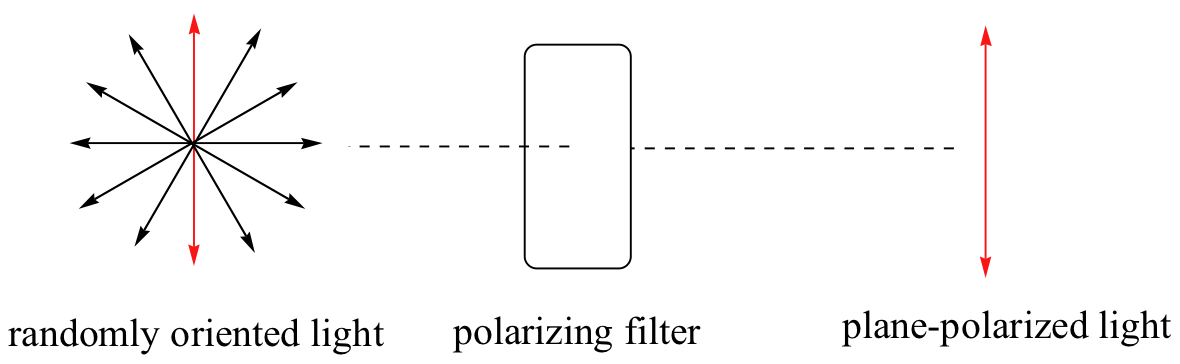# 3.6: Optical Activity

$$\newcommand{\vecs}{\overset { \rightharpoonup} {\mathbf{#1}} }$$ $$\newcommand{\vecd}{\overset{-\!-\!\rightharpoonup}{\vphantom{a}\smash {#1}}}$$$$\newcommand{\id}{\mathrm{id}}$$ $$\newcommand{\Span}{\mathrm{span}}$$ $$\newcommand{\kernel}{\mathrm{null}\,}$$ $$\newcommand{\range}{\mathrm{range}\,}$$ $$\newcommand{\RealPart}{\mathrm{Re}}$$ $$\newcommand{\ImaginaryPart}{\mathrm{Im}}$$ $$\newcommand{\Argument}{\mathrm{Arg}}$$ $$\newcommand{\norm}{\| #1 \|}$$ $$\newcommand{\inner}{\langle #1, #2 \rangle}$$ $$\newcommand{\Span}{\mathrm{span}}$$ $$\newcommand{\id}{\mathrm{id}}$$ $$\newcommand{\Span}{\mathrm{span}}$$ $$\newcommand{\kernel}{\mathrm{null}\,}$$ $$\newcommand{\range}{\mathrm{range}\,}$$ $$\newcommand{\RealPart}{\mathrm{Re}}$$ $$\newcommand{\ImaginaryPart}{\mathrm{Im}}$$ $$\newcommand{\Argument}{\mathrm{Arg}}$$ $$\newcommand{\norm}{\| #1 \|}$$ $$\newcommand{\inner}{\langle #1, #2 \rangle}$$ $$\newcommand{\Span}{\mathrm{span}}$$$$\newcommand{\AA}{\unicode[.8,0]{x212B}}$$

Chiral molecules, as we learned in the introduction to this chapter, have an interesting optical property. You may know from studying physics that light waves are oscillating electric and magnetic fields. In ordinary light, the oscillation is randomly oriented in an infinite number of planes. When ordinary light is passed through a polarizer, all planes of oscillation are filtered out except one, resulting in plane-polarized light.A beam of plane-polarized light, when passed through a sample of a chiral compound, interacts with the compound in such a way that the angle of oscillation will rotate. This property is called optical activity.If a compound rotates plane polarized light in the clockwise (+) direction, it is said to be dextrorotatory, while if it rotates light in the counterclockwise (-) direction it is levorotatory. (We mentioned L- and D-amino acids in the previous section: the L-amino acids are levorotatory). The magnitude of the observed optical activity is dependent on temperature, the wavelength of light used, solvent, concentration of the chiral sample, and the path length of the sample tube (path length is the length that the plane-polarized light travels through the chiral sample). Typically, optical activity measurements are made in a 1 decimeter (10 cm) path-length sample tube at 25 °C, using as a light source the so-called “D-line” from a sodium lamp, which has a wavelength of 589 nm. The specific rotation of a pure chiral compound at 25° is expressed by the expression:

$[\alpha]_{\mathrm{D}}^{25}=\frac{\alpha_{\mathrm{obs}}}{l c} \nonumber$

where $$\alpha_{obs}$$ is the observed rotation, $$l$$ is path length in decimeters, and $$c$$ is the concentration of the sample in grams per 100 mL. In other words, the specific rotation of a chiral compound is the optical rotation that is observed when 1 g of the compound is dissolved in enough of a given solvent to make 100 mL solution, and the rotation is measured in a 1 dm cuvette at 25 oC using light from a sodium lamp.

Every chiral molecule has a characteristic specific rotation, which is recorded in the chemical literature as a physical property just like melting point or density. Different enantiomers of a compound will always rotate plane-polarized light with an equal but opposite magnitude. (S)-ibuprofen, for example, has a specific rotation of +54.5o (dextrorotatory) in methanol, while (R)-ibuprofen has a specific rotation of -54.5o. There is no relationship between chiral compound's R/S designation and the direction of its specific rotation. For example, the S enantiomer of ibuprofen is dextrorotatory, but the S enantiomer of glyceraldehyde is levorotatory.

A 50:50 mixture of two enantiomers (a racemic mixture) will have no observable optical activity, because the two optical activities cancel each other out. In a structural drawing, a 'squigly' bond from a chiral center indicates a mixture of both R and S configurations.Chiral molecules are often labeled according to whether they are dextrorotatory or levorotatory as well as by their R/S designation. For example, the pure enantiomers of ibuprofen are labeled (S)-(+)-ibuprofen and (R)-(-)-ibuprofen, while (±)-ibuprofen refers to the racemic mixture, which is the form in which the drug is sold to consumers.

##### Exercise 3.14

The specific rotation of (R)-limonene is +11.5o in ethanol. What is the expected observed rotation of a sample of 6.00 g (S)-limonene dissolved in ethanol to a total volume of 80.0 mL in a 1.00 dm (10.0 cm) pathlength cuvette?

Exercise 3.15

The specific rotation of (S)-carvone is +61°, measured 'neat' (pure liquid sample, no solvent). The optical rotation of a mixture of R and S carvone is measured at -23°. Which enantiomer is in excess in the mixture?

Solutions to exercises

All of the twenty natural amino acids except glycine have a chiral center at their alpha-carbon (recall that basic amino acid structure and terminology was introduced in section 1.3). Virtually all of the amino acids found in nature, both in the form of free amino acids or incorporated into peptides and proteins, have what is referred to in the biochemical literature as the 'L' configuration:The 'L' indicates that these amino acid stereoisomers are levorotatory. All but one of the 19 L-amino acids have S stereochemistry at the a-carbon, using the rules of the R/S naming system.

D-amino acids (the D stands for dextrorotatory) are very rare in nature, but we will learn about an interesting example of a peptide containing one D-amino acid residue later in chapter 12.

##### Exercise 3.16

Which of the 20 common L-amino acids found in nature has the R configuration? Refer to the amino acid table for structures.

Solutions to exercises

Khan Academy video tutorial on optical acitivity

This page titled 3.6: Optical Activity is shared under a CC BY-NC-SA 4.0 license and was authored, remixed, and/or curated by via source content that was edited to the style and standards of the LibreTexts platform; a detailed edit history is available upon request.

This page titled 3.6: Optical Activity is shared under a CC BY-NC-SA 4.0 license and was authored, remixed, and/or curated by Tim Soderberg via source content that was edited to the style and standards of the LibreTexts platform; a detailed edit history is available upon request.